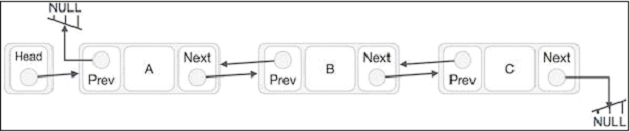# Doubly linked lists in Javascript

Doubly linked lists are represented as −Note that in the class itself, we also need to keep track of the tail(last element).

## Example

In this example, we understand the implementation of doubly linked list and its operations in JavaScript. Here, we create the list while inserting the data into it; and possibly delete nodes while removing the data from them. We are also printing the list using a user defined method print().

<!DOCTYPE html>
<html lang="en">
<meta charset="UTF-8" />
<meta http-equiv="X-UA-Compatible" content="IE=edge" />
<meta name="viewport" content="width=device-width, initial-scale=1.0" />
<body>
<script type="text/javascript">
function createNode(value) {
return {
value: value,
next: null,
previous: null,
};
}
constructor() {
this.tail = null;
this.size = 0;
}
insert(value) {
this.size++;
let newNode = createNode(value);
if (this.tail) {
this.tail.next = newNode;
newNode.previous = this.tail;
this.tail = newNode;
return newNode;
}
return newNode;
}
remove() {
if (this.tail) {
this.size--;
let removedTail = this.tail;
let beforeTail = this.tail.previous;
this.tail = beforeTail;
if (this.tail) {
this.tail.next = null;
} else {
}
return removedTail;
}
return undefined;
}
print() {
document.write("The Elements in the Doubly Linked List are :</br> ");
while (current) {
document.write(
${current.previous?.value}${current.value}   \${current.next?.value}
);
current = current.next;
}
}
this.size++;
let newNode = createNode(value);
return newNode;
}
return newNode;
}
insertIndex(value, index) {
if (index >= this.size) {
throw new Error("Insert index out of bounds");
}
if (index === 0) {
}
this.size++;
for (let i = 0; i < index; i++) {
currentNode = currentNode.next;
}
let previousNode = currentNode.previous;
let newNode = createNode(value);
newNode.next = currentNode;
newNode.previous = previousNode;
previousNode.next = newNode;
currentNode.previous = newNode;
return newNode;
}
}